• c++ matlab接口转换
2022-03-29 11:41:38

由于matlab的运算速度较慢，因此常常需要使用c++对关键部分进行运算。Matlab针对这一需求提供了相应的api方便参数传递以及封装。

这里使用的vs工程，对文件进行修改生成.mexw64文件，对于matlab可识别的文件。

这里的操作都是针对vs进行配置和操作。

## 一.工程配置

### 1.配置生成文件

右键->属性->常规->配置类型 动态库(.dll)

右键->属性->高级->目标文件扩展名 .mexw64

### 2.配置附加目录

右键->属性->c++->附加包含目录  添加$(Matlab_Dir)\extern\include; 其中$(Matlab_Dir)为matlab安装地址

右键->属性->链接器->附加库目录 添加$(Matlab_Dir)\extern\lib\win64\microsoft;; 其中$(Matlab_Dir)为matlab安装地址

### 3.添加附加依赖项

右键->属性->链接器->输入->附加依赖项

输入libmx.lib;libmex.lib;libmat.lib;libeng.lib;

## 二.接口编写

### 1.添加头文件

#include "mex.h"是matlab提供的头文件，利用提供的api进行接口函数编写

### 2.接口函数编写

入口过程的名称必须是mexFunction，并且包含四个参数

void mexFunction(int nlhs, mxArray* plhs[], int nrhs, const mxArray* prhs[]);

nlhs 输出参数个数，plhs 输出参数指针

nrhs 输入参数个数，prhs 输入参数指针

注意: 输出和输入参数的操作都是通过指针的方式进行的MATLAB可以通过这些指针，访问内存中的数据

### 3.输入参数获取

接口过程要把参数传递给计算过程，还需要从prhs中读出矩阵的信息，这就要用到下面的mx-函数和mex-函数。

• mxGetM(prhs); 获取其行数
• mxGetN(prhs); 获取其列数
• mxGetNumberOfDimensions(prhs);获取其维度

主要介绍的是数据获取的两个函数 mxGetData mxGetPr。

mxGetData: 返回值为void类型的指针，必须转换为正确数据类型的指针的指针。

mxGetPr：返回值为double类型的指针，可以理解为它会自动转换 mxGetData的输出作为double指针。
在使用上这两种都可以获取参数内容。

还有一点需要注意的是，无法对未获取的值进行操作。

下面的代码在调试时会报错，无法对于未保存的变量进行操作。

Number = ((double*)mxGetData(mxGetCell(prhs, 19)))-1;

### 4.出错信息发布函数mexErrMsgTxt，mexWarnMsgTxt

两函数的具体格式如下：

#include "mex.h"

void mexErrMsgTxt(const char *error_msg);

void mexWarnMsgTxt(const char *warning_msg);

其中error_msg包含了要显示错误信息，warning_msg包含要显示的警告信息。两函数的区别在于mexErrMsgTxt显示出错信息后即返回到MATLAB，而mexWarnMsgTxt显示警告信息后继续执行。

### 5.输出参数设置

对于程序的返回结果需要将其保存在plhs指针当中，而且保存在plhs的返回指针类型必须是mxArray。

这里介绍两个api进行数组，矩阵的创建。由于常用的数据类型为double,这里列举的均为double类型的数组。

plhs = mxCreateNumericArray(3, dims,mxDOUBLE_CLASS, mxREAL);
plhs = mxCreateDoubleMatrix(n, n, mxREAL);    

### 6.参数转置

由于matlab的数据存储顺序与c++不同，因此在数据输入时需要对数据进行转换。在数据输出传递的时候，同样也需要对数组进行转换。

matlab对于数组存储是按照列进行保存的，而c++是按照行进行保存，因此在数据计算时需要格外注意。

这里是常用的行列转换的代码。

template <class T1, class T2>
void cTranspose3d(T1* dst, T2* src, int srcRow, int srcCol, int channel)
{
int i = 0, k = 0;
T1* dst1 = NULL;
T2* src1 = NULL;

for (k = 0; k < channel; k++)
{
dst1 = dst + k * srcRow * srcCol;
src1 = src + k * srcRow * srcCol;
for (i = 0; i < srcRow * srcCol; i++)
{
dst1[i] = src1[(i % srcRow) * srcCol + (i / srcRow)];//th/ srcRow//src's  col -> dst's row,th%srcRow//src's   row -> dst's col
}
}
}


## 总结

至此整个接口书写就结束了。该篇教程也是记录一下自己整个学习过程，希望能够看的人一点点帮助。

更多相关内容
• Visual C++ MATLAB图像处理与识别实用案例精选源码.rar
• matlab打包生成的封装文件只传了三个用到的。实例demo.cpp运行正确，可供参考。
• 《visual c++matlab图像处理与识别实用案例精选》书中所有例程的源码，非常适合于书配套使用。图像处理
• 进行MATLABC++混合编程程序代码，可以用的，具体的解释参见这篇博客。https://blog.csdn.net/yanxiaoyunsana/article/details/89195238
• 版本tdm64-gcc-9.2.0，安装步骤自行百度
• MATLAB无疑是一款强大的矩阵运算与数据分析软件，内部集成了众多函数。如果在C++编程时可以调用相关的函数将对我们的数据分析研究带来极大的方便。这里就总结一下如何在VS工程中调用MATLAB函数，以及遇到的问题如何...

## 引言

MATLAB无疑是一款强大的矩阵运算与数据分析软件，内部集成了众多函数。如果在C++编程时可以调用相关的函数将对我们的数据分析研究带来极大的方便。这里就总结一下如何在VS工程中调用MATLAB函数，以及遇到的问题如何解决的方法。

## VS配置

这一步很重要，类似于其他C++库的配置，如：添加包含目录和库路径。
添加库目录（自己的MATLAB安装路径中查找）：D:\Program Files (x86)\Matlab2018a\extern\include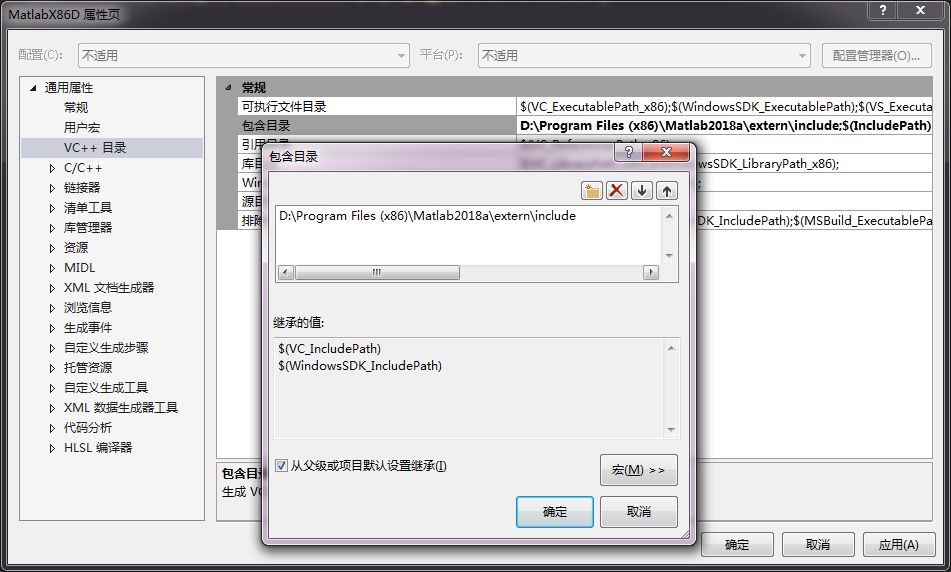添加库路径（自己的MATLAB安装路径中查找）：D:\Program Files (x86)\Matlab2018a\extern\lib\win64\microsoft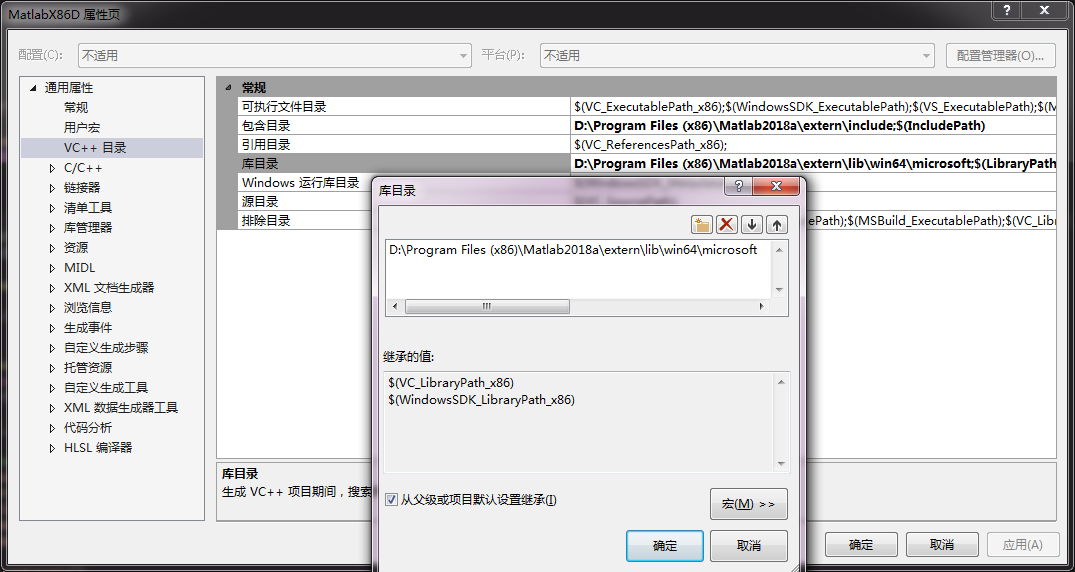## 配置系统环境变量

在Windows环境变量中的系统变量中“Path”中新增下面三项：
D:\Program Files (x86)\Matlab2018a\bin; （安装MATLAB后已经存在不用再添加）
D:\Program Files (x86)\Matlab2018a\bin\win32;
D:\Program Files (x86)\Matlab2018a\bin\win64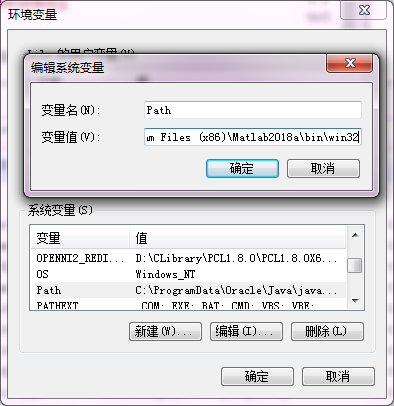## MATLAB配置

在Matlab的command window输入：mex -setup
选择：mex -setup C++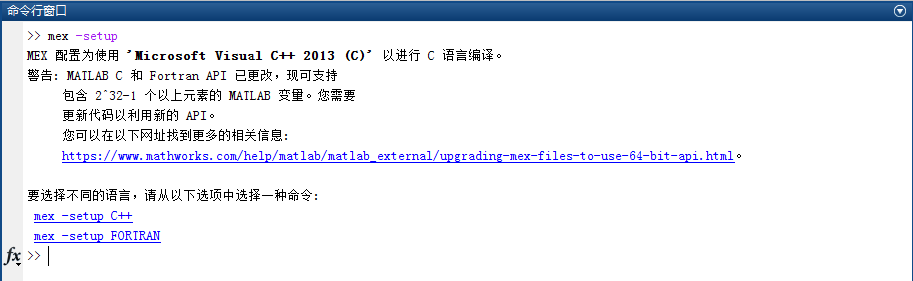然后再输入：mbuild -setup
选择：mex -setup C++ -client MBUILD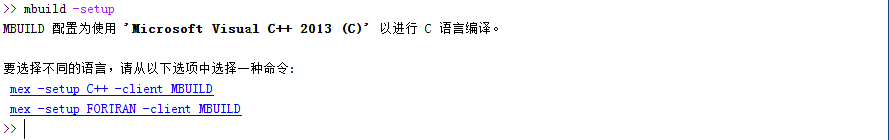配置结束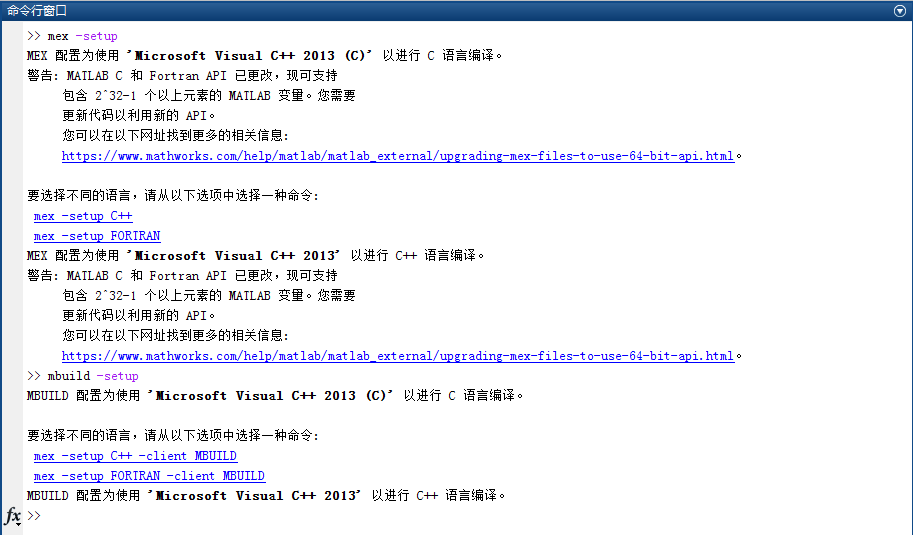## 测试

我们将在VS2013 的C++项目中调用MATLAB，调用方式有两种：（1）调用MATLAB引擎； （2）调用MATLAB函数生成的DLL。

### 方式1调用MATLAB引擎

首先，添加头文件 #include “engine.h”
然后，引用函数调用依赖库(lib) libeng.lib libmx.lib libmat.lib，之后就可以调用MATLAB引擎。

// import necessary lib
#pragma comment( lib, "libeng.lib")
#pragma comment( lib, "libmx.lib")
#pragma comment( lib, "libmat.lib")

MATLAB引擎调用和文件操作类似：打开、关闭操作。
打开引擎：

Engine *ep;
if (!(ep = engOpen("\0")))
{
fprintf(stderr, "\nCan't start MATLAB engine\n");
return EXIT_FAILURE;
} 

定义变量需要转换为matlab的格式。比如使用：mxCreateDoubleMatrix 和 memcpy函数。
将数据传入引擎使用：engPutVariable()。接着调用 engEvalString(ep, “matlab指令”)matlab的指令作为参数进行操作。计算操作等完成后需要清理操作：mxDestroyArray()函数清理mxCreateDoubleMatrix 生成的变量。
engClose()关闭引擎。

完整绘图例子：


#include <stdlib.h>
#include <stdio.h>
#include <string.h>

// import necessary lib
#pragma comment( lib, "libeng.lib")
#pragma comment( lib, "libmx.lib")
#pragma comment( lib, "libmat.lib")

int main(void)
{
Engine *ep;

// open engine
if (!(ep = engOpen("\0")))
{
fprintf(stderr, "\nCan't start MATLAB engine\n");
return EXIT_FAILURE;
}

// generate variables
int Nsample = 50;
const double PI = 3.1415926;
double *t = new double[Nsample];

for (int i = 0; i < Nsample; i++)
{
t[i] = i * 2 * PI / Nsample;
}

mxArray *T = NULL, *result = NULL;
T = mxCreateDoubleMatrix(1, Nsample, mxREAL);
memcpy((void *)mxGetPr(T), (void *)t, Nsample*sizeof(t));

engPutVariable(ep, "T", T);         // put data to engine

// execute matlab operations
engEvalString(ep, "Y=sin(T);");
engEvalString(ep, "plot(T,Y);");
engEvalString(ep, "title('y=sin(t)');");
engEvalString(ep, "xlabel('t');");
engEvalString(ep, "ylabel('y');");

fgetc(stdin);

// clean operation(don't forget!!!)
mxDestroyArray(T);
engEvalString(ep, "close;");

// close engine
engClose(ep);

return EXIT_SUCCESS;
}

效果：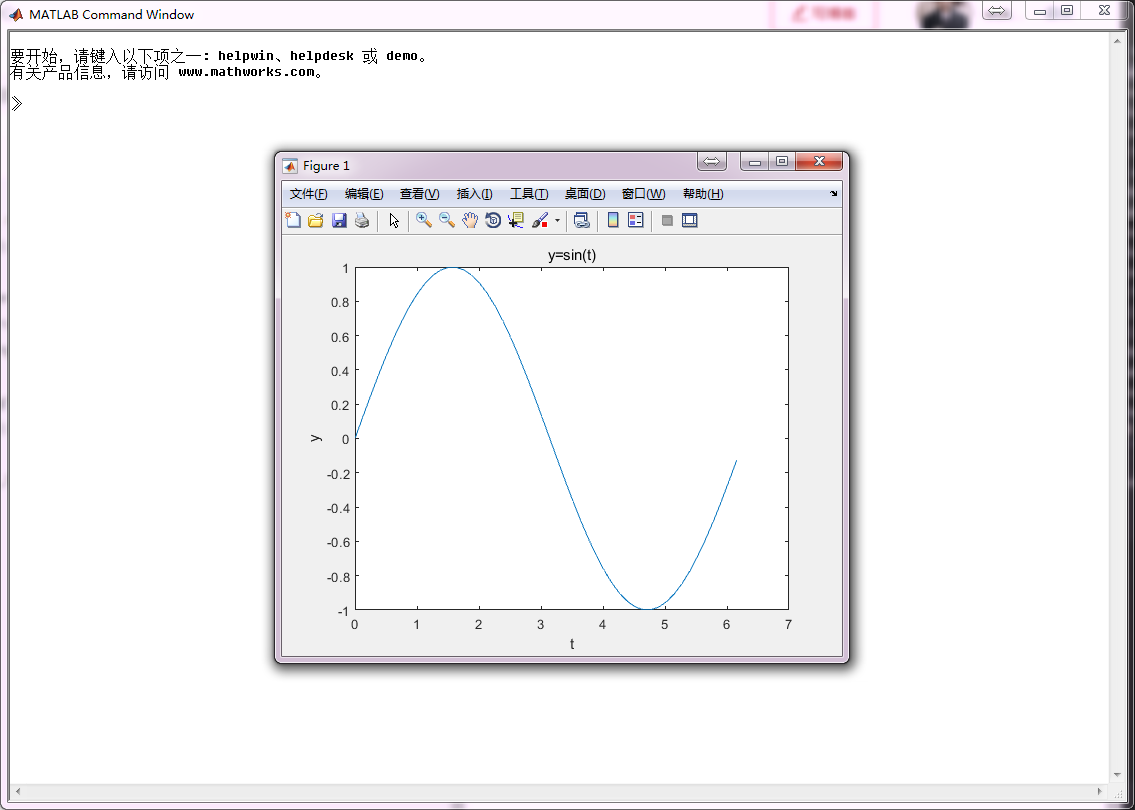出现错误解决方法：

1>------ 已启动生成:  项目: CMatlab, 配置: Debug Win32 ------
1>main.obj : error LNK2019: 无法解析的外部符号 _mxGetPr_800，该符号在函数 _main 中被引用
1>main.obj : error LNK2019: 无法解析的外部符号 _mxDestroyArray_800，该符号在函数 _main 中被引用
1>main.obj : error LNK2019: 无法解析的外部符号 _mxCreateDoubleMatrix_800，该符号在函数 _main 中被引用
1>main.obj : error LNK2019: 无法解析的外部符号 _engEvalString，该符号在函数 _main 中被引用
1>main.obj : error LNK2019: 无法解析的外部符号 _engOpen，该符号在函数 _main 中被引用
1>main.obj : error LNK2019: 无法解析的外部符号 _engClose，该符号在函数 _main 中被引用
1>main.obj : error LNK2019: 无法解析的外部符号 _engPutVariable，该符号在函数 _main 中被引用
1>E:\shizhenwei\VS2013Projects\CMatlab\Debug\CMatlab.exe : fatal error LNK1120: 7 个无法解析的外部命令

这个问题出在兼容上。我们需要将vs工程变为64位，然后即可解决。

### 方式2调用MATLAB生成的dll

调用MATLAB生成的DLL是一种比较通用的方法，这样每次使用时只需调用这个模块就可以了。
首先，将需要MATLAB完成的功能做成一个MATLAB函数。
然后，利用mcc命令编译，得到h、dll、lib等文件，在C/C++工程中只需加入上述文件即可。

MATLAB函数代码

function showBER(SNR_indB,BER)
semilogy(SNR_indB,BER,'-o','linewidth',2);
grid on
xlabel('E_b/N_0(dB)');
ylabel('BER');

编译后，在MATLAB命令行中输入：

mcc -B csharedlib:showBER showBER.m

得到一组文件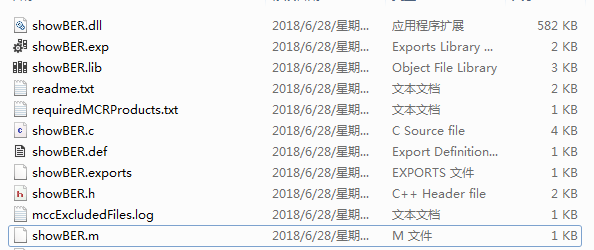将其中的.dll .h .lib文件拷贝到C工程中，在需要调用绘图模块的cpp文件中加入如下代码：

#include "showBER.h"

#pragma comment( lib, "libeng.lib" )
#pragma comment( lib, "libmx.lib" )
#pragma comment( lib, "libmat.lib" )
#pragma comment( lib, "mclmcrrt.lib" )
#pragma comment( lib, "showBER.lib" )

void main(void)
{
double SNR[] = { 0.5, 0.6, 0.7, 0.8, 0.9, 1.0, 1.1, 1.2, 1.3, 1.4, 1.5 };
double BER[] = { 9.728816e-002, 8.099609e-002, 5.633803e-002, 3.733608e-002, 1.253970e-002, 3.936489e-003, 1.206820e-003, 2.104052e-004, 3.109879e-005, 3.365857e-006, 2.565067e-007 };
int len = sizeof(SNR) / sizeof(SNR);

showBERInitialize();

mxArray* xSNR = mxCreateDoubleMatrix(1, len, mxREAL);
memcpy(mxGetPr(xSNR), (void*)SNR, sizeof(SNR));
mxArray* xBER = mxCreateDoubleMatrix(1, len, mxREAL);
memcpy(mxGetPr(xBER), (void*)BER, sizeof(BER));

mlfShowBER(xSNR, xBER);
system("PAUSE");

showBERTerminate();
}

运行效果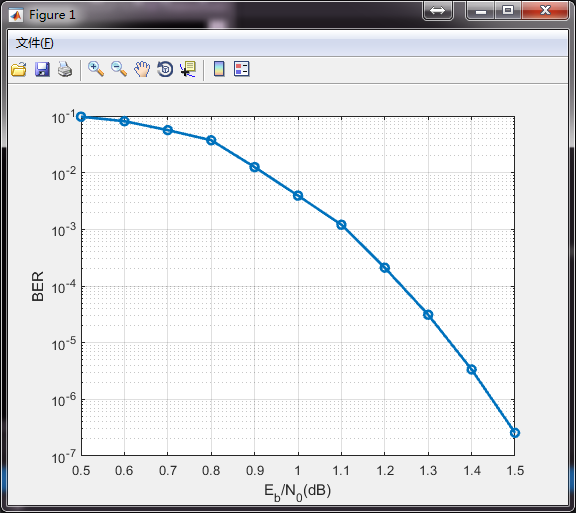### 提示

其他打包方法可以参考3和4链接中的介绍，亲测可用。

展开全文• Visual C++MATLAB图像处理与识别实用案例精选(PDG版+源代码)，包括书籍和源码两部分
• 图像处理识别方面的教程，详细介绍了图像处理的基本理论知识和matlab实现程序
• 之前已经跟大家介绍了在ubuntu系统下C++调用matlab程序的方法，需要的朋友们可以参考这篇文章，本文将给大家介绍关于windows下C++调用matlab程序的相关内容，分享出来供大家参考学习，下面话不多说了，来一起看看...
• [MATLAB]C++调用MATLAB引擎 MATLAB版本：R2018b 操作系统：Window 10 1 需求分析 在C++开发过程中，可能需要C++MATLAB混合编程，比如：别人只会MATLAB，需要和我们的C++一起运行；或者有部分MATLAB/Simulink开发...

# [MATLAB]C++调用MATLAB引擎

• MATLAB版本：R2018b
• 操作系统：Windows 10

## 2 C++/MATLAB混合编程方式

MATALB官方提供了多种MATLAB与其他语言混合编程的方法，对于C++与MATLAB的混合编程包括下面两种：

1. C++调用MATLAB
• 用于C++的MATLAB引擎API
• 将MATLAB函数编译为C++动态库
1. MATLAB调用C/C++
• MATLAB调用C++ MEX函数
• 通过calllib调用C动态库

MATLAB引擎API主要通过matlab::engine::MATLABEngine类和matlab::data::Array等类完成MATLAB的接口功能，其实C++ MEX函数本质上也是调用MATLAB引擎API的接口。后面将介绍以C++最为主程序，调用MATLAB引擎的方法。

## 3 API基础认识

这里先列举常用的类及其常用函数接口：

1. matlab::engine::MATLABEngine引擎类
• startMATLAB — 打开一个MATALB引擎
• connectMATLAB — 连接到一个已共享的MATALB引擎
• feval — 执行函数
• eval — 执行指令语句
• getVariable — 获取变量
• setVariable — 设置变量
1. matlab::data::ArrayFactory 矩阵工厂类
• createArray — 创建数组
• createScalar — 创建标量
• createCellArray — 创建元胞数组
• createCharArray — 创建Char数组
• createStructArray — 创建结构体数组
1. matlab::data::Array基础数据类型
• operator — 索引操作
• getType — 获取数据类型
• getDimensions — 获取数据维度
• getNumberOfElements — 获取数据元素个数
• isEmpty — 数据是否为空

## 4 C++编码开发

### 4.1 环境搭建

1. 安装MATLAB，安装过程略（任何版本都可以，这里以2018b为例），并假定MATLAB_ROOT作为安装路径，方便后文描述；
2. 安装CMake，这里我主要是方便QtCreator和VS2017以上都可以使用同一个cmake工程。

### 4.2 创建工程

首先创建CMake工程，指定main.cpp文件和MATLAB头文件目录、lib文件：

创建MatlabEngine文件夹，添加CMakeLists.txt文件。粘贴下面代码后执行CMake并确认无报错：

cmake_minimum_required(VERSION 3.5)
project(MatlabEngine LANGUAGES CXX)
set(CMAKE_CXX_STANDARD 11)
set(CMAKE_CXX_STANDARD_REQUIRED ON)

add_executable(${PROJECT_NAME} main.cpp) # 设置Matlab头文件及依赖库 set(MATLAB_ROOT "D:/Program Files/MATLAB/R2018b") target_include_directories(${PROJECT_NAME} PUBLIC ${MATLAB_ROOT}/extern/include) target_link_libraries(${PROJECT_NAME} PUBLIC ${MATLAB_ROOT}/extern/lib/win64/microsoft/libMatlabEngine.lib) target_link_libraries(${PROJECT_NAME} PUBLIC ${MATLAB_ROOT}/extern/lib/win64/microsoft/libMatlabDataArray.lib)  注意第9行set(MATLAB_ROOT "D:/Program Files/MATLAB/R2018b")，需要改为MATLAB实际的安装路径。 注： 对于Windows环境，需要使用到libMatlabEngine和libMatlabDataArray这两个库，对MATLAB引擎的调用实际上是对MATLAB动态库的调用。 qmake可以通过INCLUDEPATH += $$(MATLAB_ROOT)/extern/include添加头文件目录，以及LIBS += -L$$(MATLAB_ROOT)/extern/lib/win64/microsoft -llibMatlabEngine.lib -llibMatlabDataArray.lib)添加链接库文件； vs可以通过界面菜单栏的项目 > 属性进入，然后依次C/C++ > 常规 > 附加包含目录添加头文件目录，以及1.链接器 > 附加库目录 2.链接器 > 输入 > 附加依赖项添加链接库文件。 ### 4.3 编码 添加main.cpp文件，粘贴最简单的几行代码（将会创建一个figure窗口）： #include <MatlabEngine.hpp> #include <MatlabDataArray.hpp> int main() { std::unique_ptr<matlab::engine::MATLABEngine> matlabPtr = matlab::engine::startMATLAB(); matlabPtr->eval(u"figure"); return 0; }  ### 4.4 编译执行 QtCreator和VS对CMake的执行方式不太一下，这里分开说明： • QtCreator 1. 左上角工程名右键点击执行CMake； 2. 点击左侧项目，然后在构建环境栏点击详情下拉栏，点击批量编辑，输入PATH=${PATH};matlabroot\extern\bin\win64并点击OK以完成添加环境变量。注意这里的matlabroot需要改为MATLAB的实际安装路径，比如：PATH=${PATH};D:\Program Files\MATLAB\R2018b\extern\bin\win64 3. 左下角点击运行按钮（或Ctrl+R）。 • VS2019 1. 在解决方案资源管理器窗口点击右上角在解决方案和可用视图之间切换，然后选择CMake目标视图； 2. 选中MatlabEngine(可执行文件)后右键点击添加调试配置，然后在launch.vs.json文件添加一行"env": "PATH=$(PATH);matlabroot\\extern\\bin\\win64"以完成添加环境变量。注意这里的matlabroot需要改为MATLAB的实际安装路径，比如："env": "PATH=$(PATH);D:\\Program Files\\MATLAB\\R2018b\\extern\\bin\\win64"。最终launch.vs.json内容如下： { "version": "0.2.1", "defaults": {}, "configurations": [ { "type": "default", "project": "CMakeLists.txt", "projectTarget": "MatlabEngine.exe", "name": "MatlabEngine.exe", "env": "PATH=$(PATH);D:\\Program Files\\MATLAB\\R2018b\\extern\\bin\\win64"
}
]
}

3. 工具栏处点击MatlabEngine.exe开始运行（或Ctrl+F5）。

注：

如果正常运行，将会看到MATLAB生成一个通过figure命令创建的窗口。

MATLAB运行时主要依赖libMatlabEngine.dll和libMatlabDataArray.dll两个动态库，在QtCreator里面，没有找到动态库什么也不会提示，程序会直接崩溃。而VS友好一点，会提示缺少什么库。如果嫌麻烦，可以在电脑的环境变量里面将MATLAB动态库路径添加到Path里面，不过这样所有程序都会受到环境变量的影响，不建议使用。

## 5 应用

1. 创建2行3列的32位整数数组（array_2_3())：

    matlab::data::ArrayFactory factory;
matlab::data::TypedArray<int32_t> array = factory.createArray<int32_t>({2, 3});
array = 1;
array = 2;
array = 3;
array = 4;
array = 5;
array = 6;

2. 将C++的MATLAB数据类型赋值到MATLAB工作区并使用bar命令绘制柱状图(showBar())：

    m_matlab->setVariable(u"array", array);
m_matlab->eval(u"bar(array)");

3. 从MATLAB找到数组最大值并赋值到C++（findMax())：

    m_matlab->setVariable(u"array", array);
m_matlab->eval(u"maxValue = max(array, [], 'all');");
auto tmp = m_matlab->getVariable(u"maxValue");
int max = tmp;
auto label = new QLabel;
label->setText(u8"最大值：" + QString::number(max));
label->show();

4. 执行.m文件：

4.1 创建mysum.m文件：

function [output] = mysum(inputArg1,inputArg2)
output = inputArg1 + inputArg2;
end


4.2 将mysum.m移动到exe工作路径（不是exe文件路径），或者通过path命令添加mysum目录；

4.3 执行.m文件：

    m_matlab->eval(u"sumValue = mysum(1, 2);");
auto tmp = m_matlab->getVariable(u"sumValue");
int sum = tmp;
auto label = new QLabel;
label->setText(u8"两数之和：" + QString::number(sum));
label->show();

展开全文cmake
• MATLAB与C／Visual C＋＋混合编程的实现.pdf 更多资源请点击：https://blog.csdn.net/weixin_44155966
• 本文主要讲述了C++项目调用MATLAB代码的一些原理性内容，介绍了两种可用于C++项目封装MATLAB代码的方式，并对其优缺点做了详细比较。

# 系列文章目录

【C++&MATLAB】C++调用MATLAB（一）：基本原理

【C++&MATLAB】C++调用MATLAB（二）：封装MATLAB

【C++&MATLAB】C++调用MATLAB（三）：MATLAB库C++使用

注：本文主要包括一些基本原理的解释，如果您已经了解原理或者想直接进入实例，可以直接阅读后续文章

# 目录

系列文章目录

前言

一、MATLAB是什么？

二、C++如何调用MATLAB

1.MATLAB Coder

2.Library Complier

总结

# 前言

MATLAB拥有大量的开源包可以供用户使用，相比于C++繁琐复杂，MATLAB可以帮助开发人员在算法开发过程中快速验证一些核心算法的可行性，同时在C++内使用MATLAB可以帮助开发人员节省大量的开发时间。

# 一、MATLAB是什么？

MATLAB 是数百万工程师和科学家都在使用的编程和数值计算平台，支持数据分析、算法开发和建模（官网原文，哈哈）。因为集成了大量的工具包和针对性的内存管理优化，对于研究人员，包括绝大多数的理科生来说，MATLAB是一个很好的工具型代码平台。就编程的难易程度来说，MATLAB作为由C++、C以及其他编程语言二次开发的高级语言，相比于C++其具有很强的解释性，且由于其内置函数众多，可以省去开发人员大量用于编写基础算法和通用算法的时间。

# 二、C++如何调用MATLAB

C++项目使用外部代码或者资源通常有两种方式：

1.在C++项目中直接添加代码文件（.h和.cpp文件）；

2.在C++项目中引用链接库（lib、dll库）。

那么对于MATLAB来说，它提供了对应这两种方案的两种工具包：MATLAB Coder和Library Complier来分别支持这两种方式，且这两个工具包都具有独立的UI界面（MATLAB早期的一些版本这些功能可能不存在，建议使用MATLAB2018b以后的版本）。通过这两种方式，我们可以很好的将MATLAB项目或者功能移植C++项目中。

但对于用户使用来说，这两种方式并不是等价的，可以说是各有千秋。

## 1.MATLAB Coder

MATLAB Coder可以将MATLAB语言代码和部分工具库函数进行代码转换，转换成对应C++项目文件或者打包成一个小型（只包含所需部分）的链接库。由于编程语言规范之间的差异，这种代码转换的缺点也就十分明显，在转换代码之前我们需要按照一定的C++代码编写规范去编写MATLAB代码，例如：我们需要在使用变量前对变量进行定义，这在MATLAB里面是不需要的。不过，好在MATLAB Coder在转换之前会自动检测代码规范，并在不符合规范出给予修改提示。但是如果这个不规范出现在工具库函数中，我们可能就无能为力了，只能去使用Library Complier来进行MATLAB封装。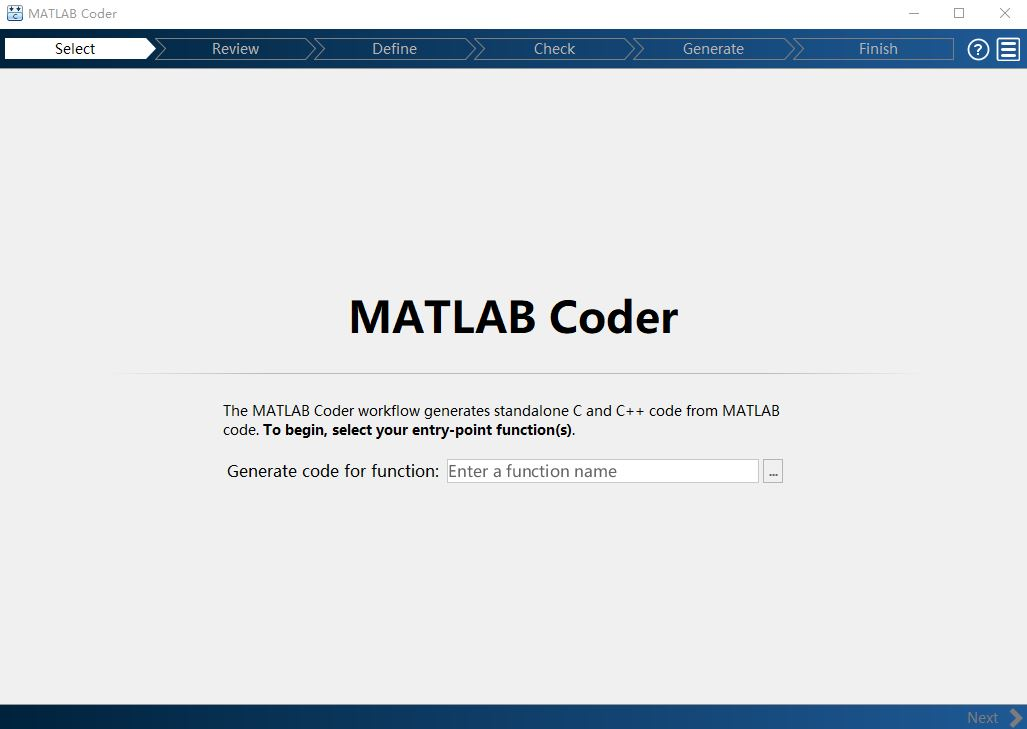## 2.Library Complier

Library Complier可以将整个MATLAB进行封装打包成为链接库，供C++项目使用。相比于MATLAB Coder，Library Complier可以无视两种语言之间的规范差异，转而将整个MATLAB进行封装，通过调用链接库内函数的方式来为C++项目处理一些棘手的问题，而我们所需要做的就是在MATLAB中编写好我们希望使用功能的API（接口函数）即可。但很显然的是，将整个MATLAB打包会增加整个项目的体积，以及降低整个项目的运行效率。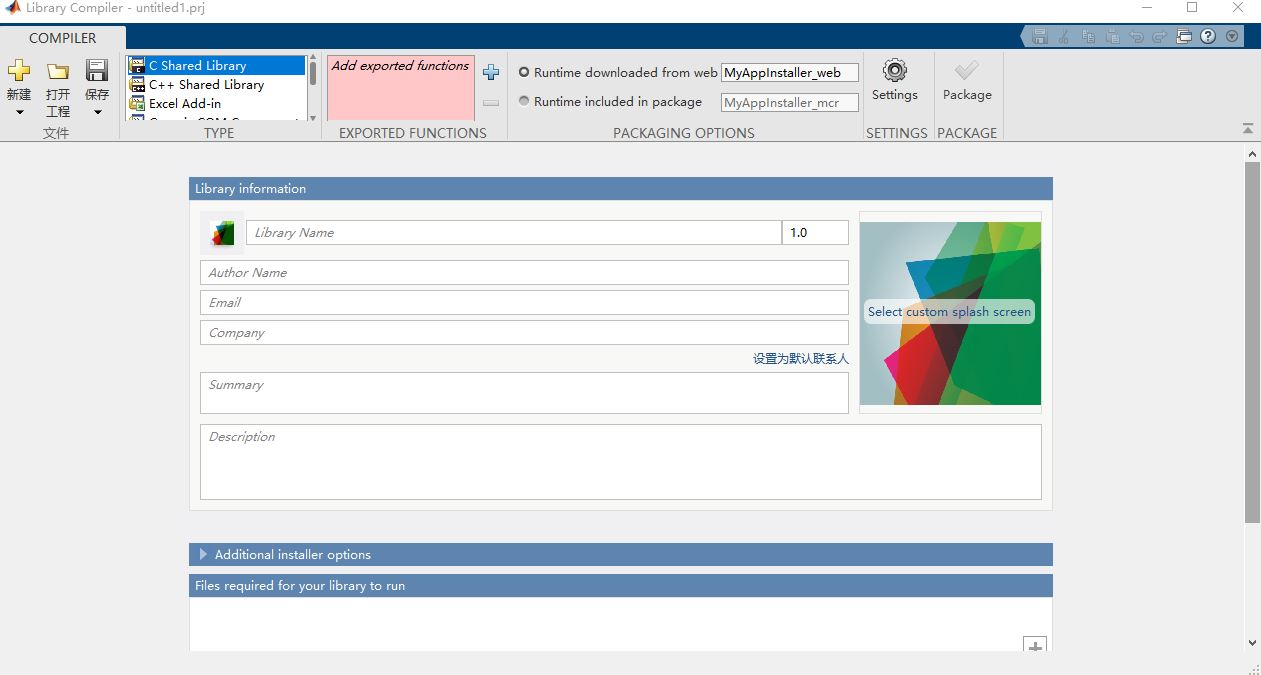总的来说MATLAB Coder与Library Complier的区别就是在于：MATLAB Coder生成的文件更加轻量化、拥有较高的效率但需要遵守部分C++编写规范且在某些MATLAB 工具库函数上无法工作；而Library Complier则适用于几乎所有MATLAB代码，但生成的文件比较笨重使用起来效率相对较低。

# 总结

本文主要讲述了C++项目调用MATLAB代码的一些原理性内容，介绍了两种可用于C++项目封装MATLAB代码的方式，并对其优缺点做了详细比较。

ps：博主初来咋到，欢迎大家对不懂的评论留言提问^_^

展开全文开发语言
• 本篇笔记将纪录C++调用Matlab封装生成的动态库。 编译环境：VS2008 + Matlab2010a 一、Matlab编译生成dll 编写一个myadd.m文件，实现加法： function [y,z]=myadd(a,b) y = a+b; z = a+2*b; end 在Matlab的...
• MinGW-w64 是基于 GNU 工具链的 Windows 编译器套件。...可以使用 MEX 从 MATLAB 调用使用 MinGW-w64 GCC 编译的 C 和 C++ 应用程序。这个 GCC 编译器对于需要 C 或 C++ 编译器的其他 MathWorks 产品也很有帮助。
• 《visual c++matlab图像处理与识别实用案例精选》课本的电子档，使用超星阅读器进行阅读。
• -> Matlab 类型转换器简介：C/C++ 类型（本机、STL、openCV...）和 Matlab 矩阵（编译或运行时）之间的双向转换。 关键词：C、C++、mxArray、OpenCV、IplImage、迭代器、mex、引擎 描述： 考虑以下场景（尤其是在...
• 《visual+c++matlab图像处理与识别实用案例精选》源码《visual+c++matlab图像处理与识别实用案例精选》源码《visual+c++matlab图像处理与识别实用案例精选》源码
• visual c++ matlab图像处理与识别实用案例精选visual c++ matlab图像处理与识别实用案例精选visual c++ matlab图像处理与识别实用案例精选
• 本文件是C++调用MATLAB的程序，平台是VS2012和MATLAB 2017，这里的平台不能随意更换，因为存在兼容性问题。程序整体已经通过测试。相关的混合编程理论可见我的博客文章。
• 本文介绍了当封装好MATLAB后，如何通过配置VS 2019环境，对MATLAB库进行调用完成Hello World!打印。
• 本文讲述了如何利用MATLAB 内置的Library Complier APP对MATLAB函数进行封装，生成可供C++项目使用的链接库及头文件
• 学习c++matlab混合编程一般是通过c++调用matlab函数，因为matlab具有强大的数学函数库，然而vc++具有界面设计灵活的优点，下面这篇文章主要给大家介绍了关于在ubuntu系统下C++调用matlab程序的方法，需要的朋友...
• 这个例子演示了通过mcc将.m函数编译成动态链接库供c++调用的方式实现c++matlab的交互。具体例子介绍请结合我的博文：blog.csdn.net/arackethis/article/details/43372553
• 本书系统地介绍了图像处理与识别的基本原理、典型方法和实用技术。全书共分12章，第1章～第6章是图像...第11章～第12章，是两个综合性较强的实例，分别是Visual C++实现的基于神经网络的文字识别系统和车牌定位系统。
• 基于C++ matlab等语言实验kalman算法代码，希望有用
• C++MatLab协作使用比较复杂，逐步学习积累记录，侧重于在C++程序中调用MatLab接口。 环境 Ubuntu 20.04 VSCode MatLab R2021a for Linux 1. C++中使用的MatLab头文件与API等 1.1头文件 MatLab自带供C/C++语言...
• 在QT中调用Matlab初始化时，出现了“函数或变量 ‘matlabrc’ 无法识别”的情况，接着崩溃。 而且比较神奇的是，前一次是可以初始化的，但运行过程中发生了崩溃。 直接搜解决办法，发现冰柚子给出了有效的解决办法...
• 该压缩包提供如何封装matlab的头文件为C++可调用的动态编辑库和两个不同输入参数类型的小demo...

# c++ matlabc++ 订阅matlab 订阅